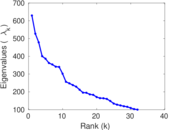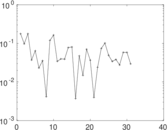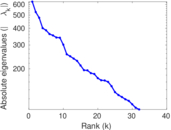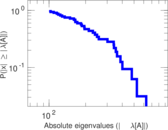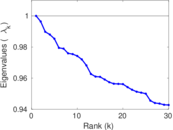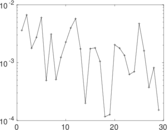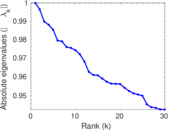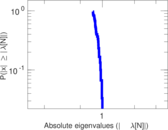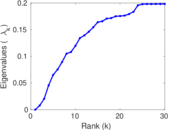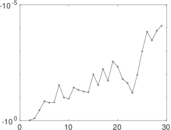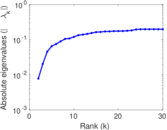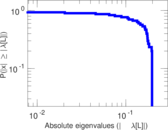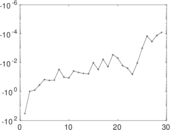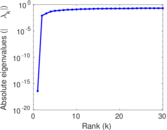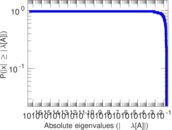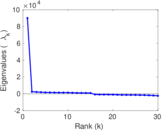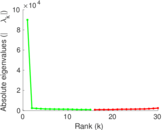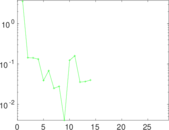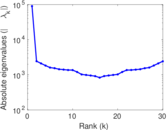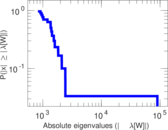# Wikipedia edits (pa)

This is the bipartite edit network of the Punjabi Wikipedia. It contains users and pages from the Punjabi Wikipedia, connected by edit events. Each edge represents an edit. The dataset includes the timestamp of each edit.

 Code `pa` Internal name `edit-pawiki` Name Wikipedia edits (pa) Data source http://dumps.wikimedia.org/ AvailabilityDataset is available for download Consistency checkDataset passed all tests Category Authorship network Dataset timestamp 2017-10-20 Node meaning User, article Edge meaning Edit Network formatBipartite, undirected Edge typeUnweighted, multiple edges Temporal dataEdges are annotated with timestamps

## Statistics

 Size n = 90,005 Left size n1 = 2,556 Right size n2 = 87,449 Volume m = 359,766 Unique edge count m̿ = 189,836 Wedge count s = 737,583,603 Claw count z = 3,433,668,557,027 Cross count x = 13,986,413,949,489,736 Square count q = 229,270,842 4-Tour count T4 = 4,784,956,640 Maximum degree dmax = 48,500 Maximum left degree d1max = 48,500 Maximum right degree d2max = 2,029 Average degree d = 7.994 36 Average left degree d1 = 140.754 Average right degree d2 = 4.114 01 Fill p = 0.000 849 303 Average edge multiplicity m̃ = 1.895 14 Size of LCC N = 88,948 Diameter δ = 13 50-Percentile effective diameter δ0.5 = 3.460 75 90-Percentile effective diameter δ0.9 = 4.037 71 Median distance δM = 4 Mean distance δm = 3.872 94 Gini coefficient G = 0.804 026 Balanced inequality ratio P = 0.173 199 Left balanced inequality ratio P1 = 0.049 724 0 Right balanced inequality ratio P2 = 0.252 764 Relative edge distribution entropy Her = 0.717 180 Power law exponent γ = 3.241 83 Tail power law exponent γt = 2.411 00 Tail power law exponent with p γ3 = 2.411 00 p-value p = 0.000 00 Left tail power law exponent with p γ3,1 = 1.551 00 Left p-value p1 = 0.000 00 Right tail power law exponent with p γ3,2 = 2.471 00 Right p-value p2 = 0.000 00 Degree assortativity ρ = −0.291 245 Degree assortativity p-value pρ = 0.000 00 Spectral norm α = 629.430 Algebraic connectivity a = 0.007 796 32 Spectral separation |λ1[A] / λ2[A]| = 1.193 72 Controllability C = 85,081 Relative controllability Cr = 0.946 891

## Plots

### Fruchterman–Reingold graph drawing### Degree distribution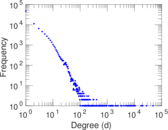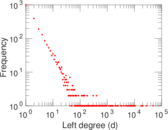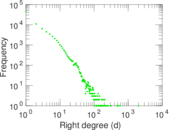### Cumulative degree distribution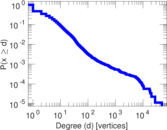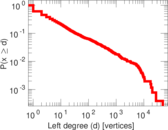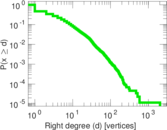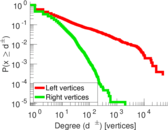### Lorenz curve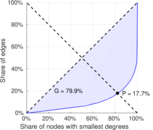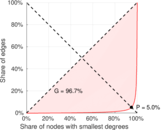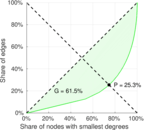### Spectral distribution of the adjacency matrix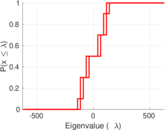### Spectral distribution of the normalized adjacency matrix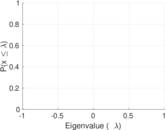### Spectral distribution of the Laplacian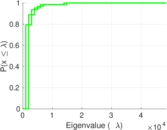### Spectral graph drawing based on the adjacency matrix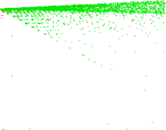### Spectral graph drawing based on the Laplacian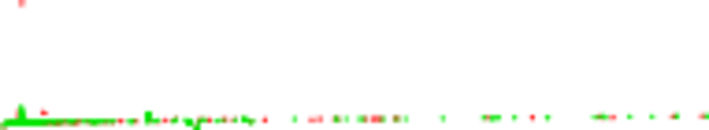### Spectral graph drawing based on the normalized adjacency matrix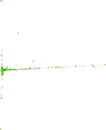### Degree assortativity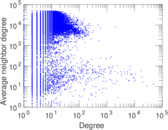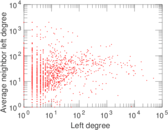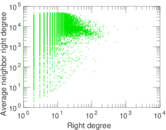### Zipf plot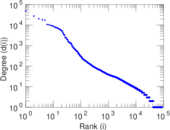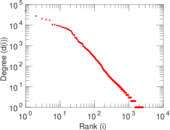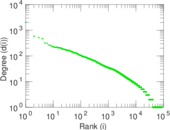### Hop distribution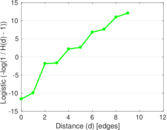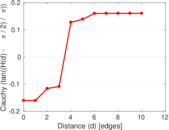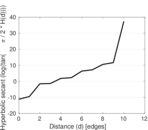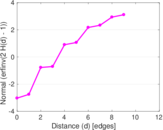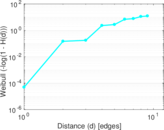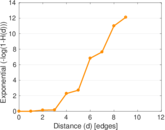### Delaunay graph drawing### Edge weight/multiplicity distribution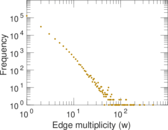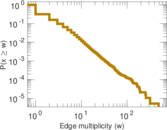### Temporal distribution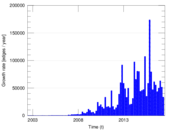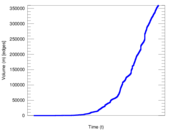### Temporal hop distribution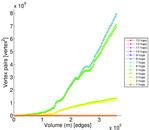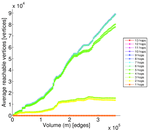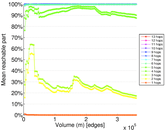### Diameter/density evolution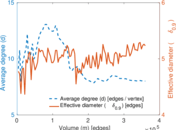### Matrix decompositions plots